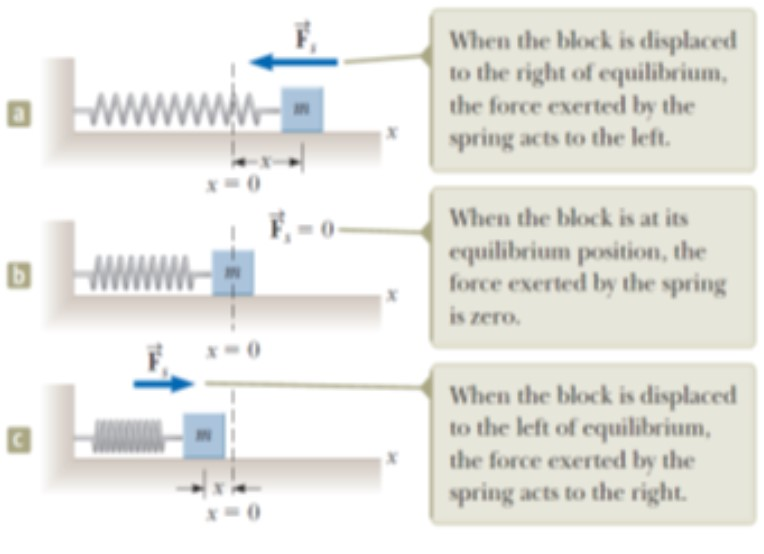USE PROMO CODE "DEMO100" TO AVAIL FREE DEMO CLASSOscillatory motion

Oscillatory motion is a type of motion of an object that regularly returns to a given position after a fixed time interval. There are several types of oscillatory motion that we observe in our life ex. A pendulum watch, spring when streched or compressed, etc.

A special kind of oscillatory motion occurs in mechanical systems when the force acting on an object is proportional to the position of the object relative to some equilibrium position. If this force is always directred toward the equilibrium position, the motion is called simple harmonic motion.

Motion of an object attached to a spring

As a model for simple harmonic motion, we consider a block of mass m attached to the end of a spring, with the block free to move on a frictionless, horizontal surface as shown in the figure below:In the above figure when the spring is neither stretched nor compressed, the block is at rest at the position called the equilibrium position of the system, which we identify as x= 0. Such a system oscillates back and forth, if disturbed from its equilibrium position.

We can understand the oscillating motion of the block in above figure by exerting a force and causing a displacement of x in the block. The exerted force is directly proportional to the displacement x of the block given by Hooke’s law.

Hook’s law: Fs = - KX

Fs – restoring force (it is always against the displacement, hence negative)

K – hook’s constant

X – displacement

## Join As Tutor Or Student

Join our community that have more than 10,000 subscribers and learn new things everyday